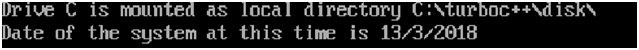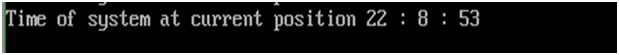# getdate() and gettime() functions of dos.h in C

In this article, we are going to learn about the process of getting the current time and date of our system by using getdate() and gettime() functions of dos.h header file in C.
Submitted by Manu Jemini, on March 15, 2018

These are very useful functions for any c programmer, as they gave the current date and time. The Usage of these functions is fairly simple, as all we need to do is, create a struct date. Pass this to the getdate() and then use it to get the day, month etc.

Similarly, when we are looking at the gettime() function, it also asks for a time object to be passed and then uses that object to get the current hour and minute etc.

The Application of these functions is numerous e.g. showing a digital clock or a calendar etc.

These functions are from the dos.h, file which should always be included in the program.

## dos.h - getdate() function Example in C

﻿

```#include <stdio.h>
//to use 'getdate()'
#include <dos.h>

int main()
{
// creating date object.
struct date a;

// getting date using function.
getdate(&a);

//printing the message with current system date.
printf("Date of the system at this time is %d/%d/%d\n",a.da_day,a.da_mon,a.da_year);

return 0;
}
```

Output## dos.h - gettime() function Example in C

﻿

```#include <stdio.h>
//to use 'gettime()'
#include <dos.h>

int main()
{
// creating time object.
struct time k;

// getting time using function.
gettime(&k);

//printing the message with current system time.
printf("Time of system at current position  %d : %d : %d\n",k.ti_hour,k.ti_min,k.ti_sec);

return 0;
}
```

Output